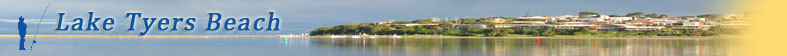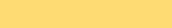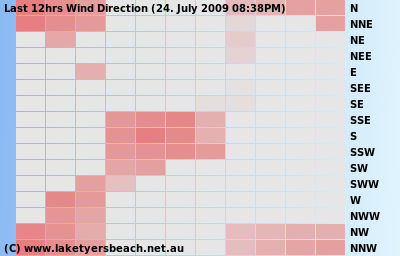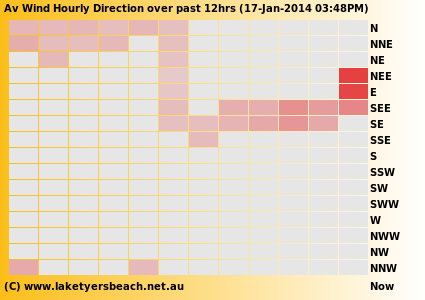# Averaging Wind Direction

 Averaging the wind direction is not quite a simple matter.  The wind direction is reported by the Wind Vane in 16 subdivisions of the compass, so from N(0), NNE(1), NE(2) through to NW(14), NNW(15) are reported.  Here is a simple hypothetical example to demonstrate the problem.  Say we have a wind direction near to NNW (15) and N(0), and we get 8 readings of NNW(15) and 8 readings of N(0).  The simple linear average of these readings  would be 7.5, and give an average direction of SSE(7), the complete opposite direction!!! (see results below) What is required is a Circular Averaging system and to do that we use the circular trigonometric functions, Sine and Cosine and ArcTangent. Working in Radians, a circle has 2pi radians.  Each of the sixteen real time readings are teated as an angle, and assigned a proportional value out of  2pi max.  eg 3/16ths of 2pi.  Then for each of the wind vane readings of 16 angles, the Sine and the Cosine of each angle read is calculated.  The results of all these Sines are summed, and the results of all the Cosines are also separately summed. Averages are calculated for both the Sines and the Cosines.  The Sines average is then divided by the Cosine average and the resulting number is turned back in the Average Angle using the ATan function. (see Program Below)This the previous map of the wind direction before averaging, showing the basic idea but more "indecisive".  It shows the spread , but instantaneous values on the weather table and published elsewhere could be highly misleading. Now the mathematicians amongst us will be bristling with a question right now: How does the ATan differentiate between a positive number returned from the division of the AvSines by the AvCosines (eg +0.4) when the angle falls in the first quadrant where Sine and Cos are always positive, and the same number (ie +0.4) but it is returned from an angle in the third quadrant where both Sin and Cos are always negative. (Reminder: Negative divided by negative equals a positive and so can return a similar +0.4 result).  The same problem exists with the negative results from  second and fourth quadrants, where Sin and Cos are always opposite signs. The computer language designers have come to our rescue and have a reverse Tan function called ATAN2.  This accepts the AvSines and AvCosines as two separate numbers and can therefore take into account the signs of the Sine and Cosine averages, and thus distinguish first and third quadrant results, as well as second and fourth quadrant results.  The Python 3 test-bed program is shown below.  ATAN2 does return a result though between -180 to +180, so you will see a little extra modulo 16 maths is required to make sure we are only ever get 0-15.A tighter graph is produced and tracks the overall changes a lot closer and handles values close to NNW-N ok. #PYTHON 3 import math myquadrant=[0,15,0,15,0,15,0,15,0,15,0,15,0,15,0,15] #Test the Cross-over point #myquadrant=[10,11,10,11,10,11,10,11,10,11,10,11,10,11,10,11] #test the double negative quadrant #myquadrant=[12,12,12,12,12,12,12,12,12,12,12,12,12,12,12,12] #test the Cosine 0 value #myquadrant=[4,4,4,4,4,4,4,4,4,4,4,4,4,4,4,4] #test the other Cosine 0 value #myquadrant=[8,8,8,8,8,8,8,8,8,8,8,8,8,8,8,8] #test the Pi value #myquadrant=[0,0,0,0,0,0,0,0,0,0,0,0,0,0,0,0] #test the 0 value #myquadrant=[15,15,15,15,15,15,15,15,15,15,15,15,15,15,15,15] #test the neares to 0 value #myquadrant=[0,0,0,0,0,0,0,0,0,0,0,0,0,0,0,15] #test the neares to 0 value #Now calculate the average wind direction using circular functions sin and cos sumSin=0.0 sumCos=0.0 sumDir=0.0 pi=math.pi pi8=math.pi/8.0 for i in range(0,16):   sumDir=sumDir+myquadrant[i]   sumSin=sumSin+math.sin(myquadrant[i]*pi8)   sumCos=sumCos+math.cos(myquadrant[i]*pi8) avSin=sumSin/16.0 avCos=sumCos/16.0 avDir=(sumDir/16.0) print ("Curent data:") print (myquadrant) print ("- - - - - - - - - - - - - - - - - - - - - - - - - - -") print ("Simple Linear Av="+str(avDir)+"/16 possibilities") print ("  Predicted Linear Av Degrees ="+str(avDir*22.5)+"/360") print ("  Predicted Radians ="+str(avDir*pi8)+"/2pi") print ("  Predicted R-Degs  ="+str(math.degrees(avDir*pi8))+"/360") print ("Calculated circular Average") print ("Av Sin="+str(avSin)+", Av Cos="+str(avCos)) print ("  Atan2="+str(math.atan2(avSin,avCos))) print ("  Degrees="+str(math.degrees((math.atan2(avSin,avCos))))) print ("  Direction# = "+str(((int(math.atan2(avSin,avCos)/pi8))+16)%16)+"/16") Here are the results: Current data: [0, 15, 0, 15, 0, 15, 0, 15, 0, 15, 0, 15, 0, 15, 0, 15] - - - - - - - - - - - - - - - - - - - - - - - - - - - Simple Linear Av=7.5/16 possibilities   Predicted Linear Av Degrees =168.75/360   Predicted Radians =2.94/2pi   Predicted R-Degs  =168.75/360 Calculated circular Average Av Sin=-0.1913, Av Cos=0.9619   Atan2=-0.1963   Degrees=-11.25   Direction# = 0/16 So due north (ie N(0) is better than 168degrees, SEE(7)!!!

If you have heard of the East Coast Lows and how they bring rain to far east Gippsland then have a look at this page.